Mathematics

# Interquartile Range

1.5k views

 1 Introduction 2 Types of data 3 Graphical Data Introduction 4 Interquartile Range 5 Steps to find IQR 6 Summary 7 FAQs 8 External References

09 November 2020

## Introduction

The interquartile range means the differences between the higher Quartile and Lower Quartile in a given set of data.

The term data means Facts or figures of something. It came from the Latin word ‘datum’ which means “something given”.

And information means knowledge of something which came from the Latin word ” Informatio “ meaning Conception.

 Difference between Data and Information Data Raw facts of things No contextual meaning Just number and text Information Data with exact Meaning Processed data

Graphical representation of data means the representation of data through figures and charts.

In this article, we will discuss the type of data, Introduction to Graphical data, and Interquartile range of data.

If you ever want to read it again as many times as you want, here is a downloadable PDF to explore more.

## Types of data

In Mathematics there are two types of data

• Continuous data
• Discrete data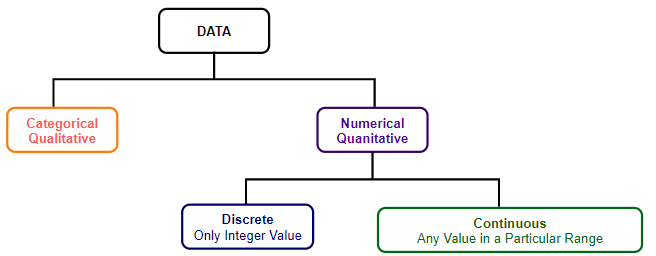### Continuous data

Continuous data is the data that can take any values. It represents the measurement and that’s why it can take any value. There are some kinds of continuous data that can be changed over time. Example height of a tree, weight of a fruit, etc.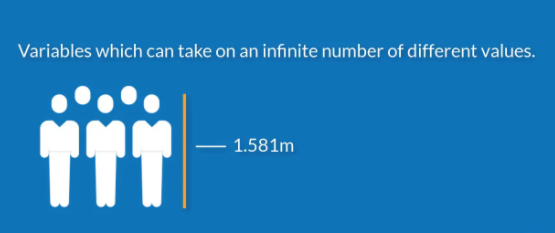### Discrete Data

This type of data can not take all values. It can contain a certain type of value so it can not be measured but only can be counted. Example: number of children in a particular grade which can’t be 33.72 or can not be measured but counted.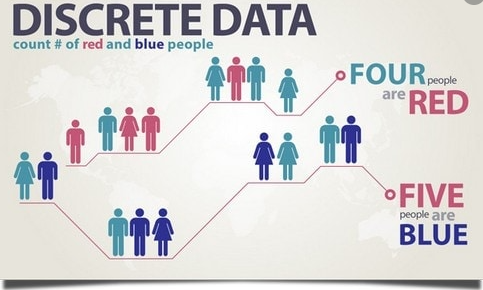### Discrete data vs Continuous data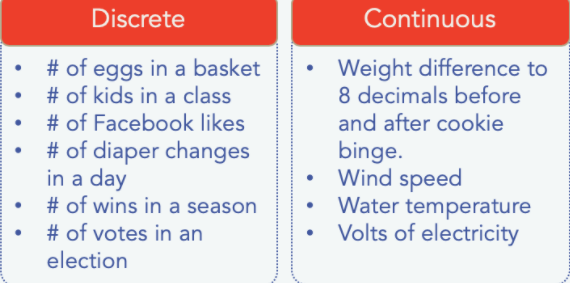## Introduction to Graphical data

It is very easy to adept and analyzes data when we can visualize it.

There are a few types of Graphs we can use to represent data. Below we will discuss the types of graphs.

### Scatter plot

This is a mathematical diagram which is drawn by using the Cartesian coordinate to represent the relationship of two variables.

Example: To show the relationship between the sales of some goods which is dependable to the temperature we can use a Scatter plot.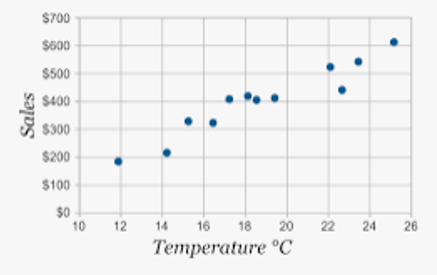### Line Plot

A-line plot or Line graph is a graph that represents a series of data by using cartesian coordinate in the form of dots or here it is called marker which is further joined by a line segment.

The line plot displays the trend of data over time.

Example: To show the trend of change in the number of species in a particular area over a time period, we can use a line plot.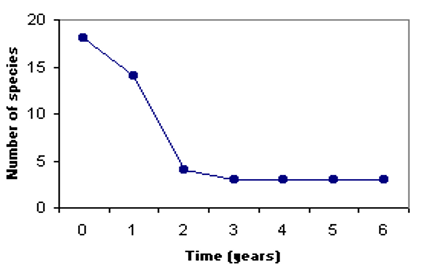### Bar chart

The bar chart or graph is the graph where categorical data is represented by using the rectangular bar.

The height or the length of the bar represents the values.

Example: To show the favorite color the children is a categorical data which can be represented by the Bar chart.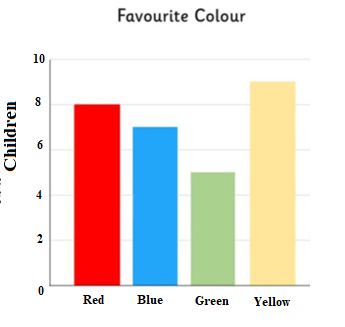### Histograms

A histogram is a type of chart or graph where data are represented by a rectangular bar and the height or length of the bar is proportional to the values of the data.

Though the bar chart and histogram looks similar, it is different as the bar chart represents the categorical value where the histogram represents numerical ranges.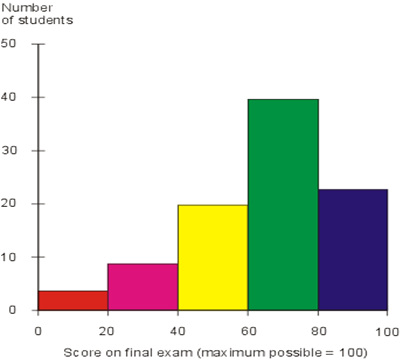### Pie chart

A pie chart is a circular chart where values are represented as the slice according to their numerical proportion.

The whole circle represents 100% where the slices are a fractional form of the data.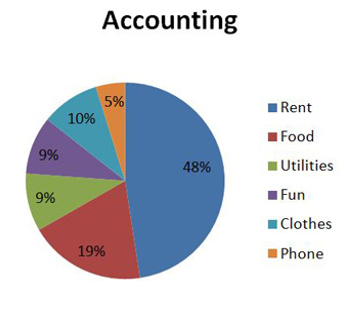## Interquartile range

Quartile means a quantile that divides a ranked data into four equal parts.

And the Interquartile range is the distance between the highest or upper quartile and the lowest or lower quartile.

It is called IQR and used as the measure of variability in a given data set.

The Quartile which divides a given data set into four equal parts is known as the First quartile- Q1, second quartile- Q2, and Third quartile- Q3 respectively.

• Q1 is the mid-value of the first half.
• Q2 is the Median
• Q3 is the mid-value of the second half or last half.

The Formula of Interquartile range is

### Even Sample space

When the sample is even number (n=10) the middle value or the median is after the first 5 values and after the median value, there are 5 more values.

In the given sample data below the median value is 71.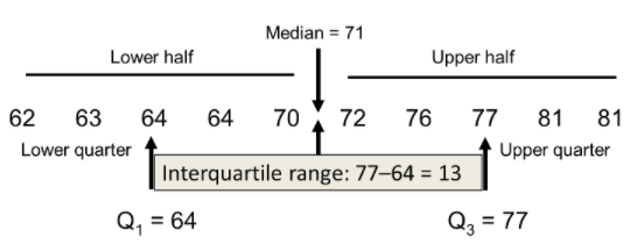So we can say that 50% of the value is above 71 and 50% after.

The mid-value of the first quartile 64 and 77 is the mid-value of the third quartile.

So the difference between the third quartile and the first quartile is 77-64= 13.

### Odd sample space

When the number of samples is odd (n=9), the median value is the middle one that is 5th one, or in this data given below 72 is the median value.

The Lower quartile or 1st quartile has four values as well as the higher quartile or 3rd quartile has four values.

So Q1= 64+64/2=64 and Q3= 77+81/= 79.

Therefore Interquartile range= 79-64= 15.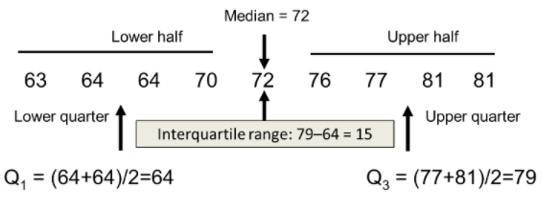## Steps to find IQR

The steps are,

• Step 1: We have to arrange the data in ascending order.
• Step 2: We have to find the median. Now if the sample is of even number then the median is the average of two middle points and if the sample number is odd then the median is the middle point of the data.
• Step 3: Then find the first quartile. The 1st quartile or Q1is the median of the lower half which is on the left side of the median of the given set of data.
• Step 4: Next is to find the third quartile or Q3. Q3 is the median of the upper quarter and is on the right side of the median value of the given data set.
• Step 5: Now to find the Interquartile range we have to Subtract Q1 from Q3.

### Example

In an essay competition, maks are given on a 6 point scale. Find the IQR of the given data, $$1,3,3,3,4,4,4,6,6$$

• Step 1: The given set of data is arranged in ascending order.

• Step 2: As the sample number is odd so the median value is 4.

• Step 3: Q1 is the median of 1st half of the data on the left side of the Median.

1 3 3 3, which is $$3+\frac{3}{2} = 3.$$

• Step 4: Q3 is the median of 2nd quarter of the data on the right side of the Median.

$$4 \; 4 \;6 \; 6,$$which is $$4+\frac{6}{2}=5.$$

• Step 5: So the Interquartile range of data is Q3-Q1= 5-3=2.

## Summary

An interquartile range of data is the way to find the middle 50% of a given data set.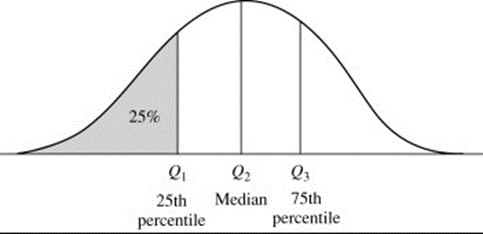The range of a given set is the difference between the maximum value and the minimum value.

The Interquartile range gives us the measurement, how data is spread out in a given data set.

The most significant point of using IQR is that it is resistant to the outlier.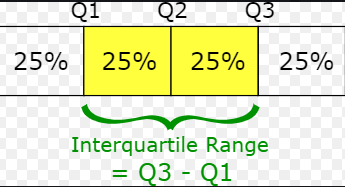Cuemath, a student-friendly mathematics platform, conducts regular Online Live Classes for academics and skill-development and their Mental Math App, on both iOS and Android, is a one-stop solution for kids to develop multiple skills. Know more about the Cuemath fee here, Cuemath Fee

## What is data?

Data is a collection of facts and statistics for analysis.

## How do you differentiate between data and information?

Data is a collection of facts. Whereas, information is how you understand those facts.

## What are the ways to represent data?

Data can be represented using graphs, tables and charts.

## What are the different types of graphs?

Various types of graphs are bar graph, line graph, pictograph etc.

## How to find the interquartile range?

The steps to find IQR are,

• Step 1: We have to arrange the data in ascending order.
• Step 2: We have to find the median. Now if the sample is of even number then the median is the average of two middle points and if the sample number is odd then the median is the middle point of the data.
• Step 3: Then find the first quartile. The 1st quartile or Q1is the median of the lower half which is on the left side of the median of the given set of data.
• Step 4: Next is to find the third quartile or Q3. Q3 is the median of the upper quarter and is on the right side of the median value of the given data set.
• Step 5: Now to find the Interquartile range we have to Subtract Q1 from Q3.

## What is the interquartile range?

Quartile means a quantile that divides a ranked data into four equal parts. And the Interquartile range is the distance between the highest or upper quartile and the lowest or lower quartile. It is called IQR and used as the measure of variability in a given data set.

## External References

To know more about interquartile range visit:

Where do we use interquartile range?

Award-winning math curriculum, FREE for a year